### IMO Shortlist 1994 problem N4

Kvaliteta:
Avg: 0,0
Težina:
Avg: 7,0
Define the sequences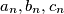$a_n, b_n, c_n$ as follows.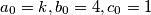$a_0 = k, b_0 = 4, c_0 = 1$.
If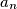$a_n$ is even then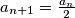$a_{n + 1} = \frac {a_n}{2}$,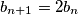$b_{n + 1} = 2b_n$,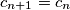$c_{n + 1} = c_n$.
If$a_n$ is odd, then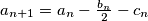$a_{n + 1} = a_n - \frac {b_n}{2} - c_n$,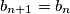$b_{n + 1} = b_n$,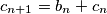$c_{n + 1} = b_n + c_n$.
Find the number of positive integers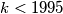$k < 1995$ such that some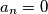$a_n = 0$.
Izvor: Međunarodna matematička olimpijada, shortlist 1994